Check Important Questions and Chapter Summary -https://you.tube/Real-Numbers-Class-10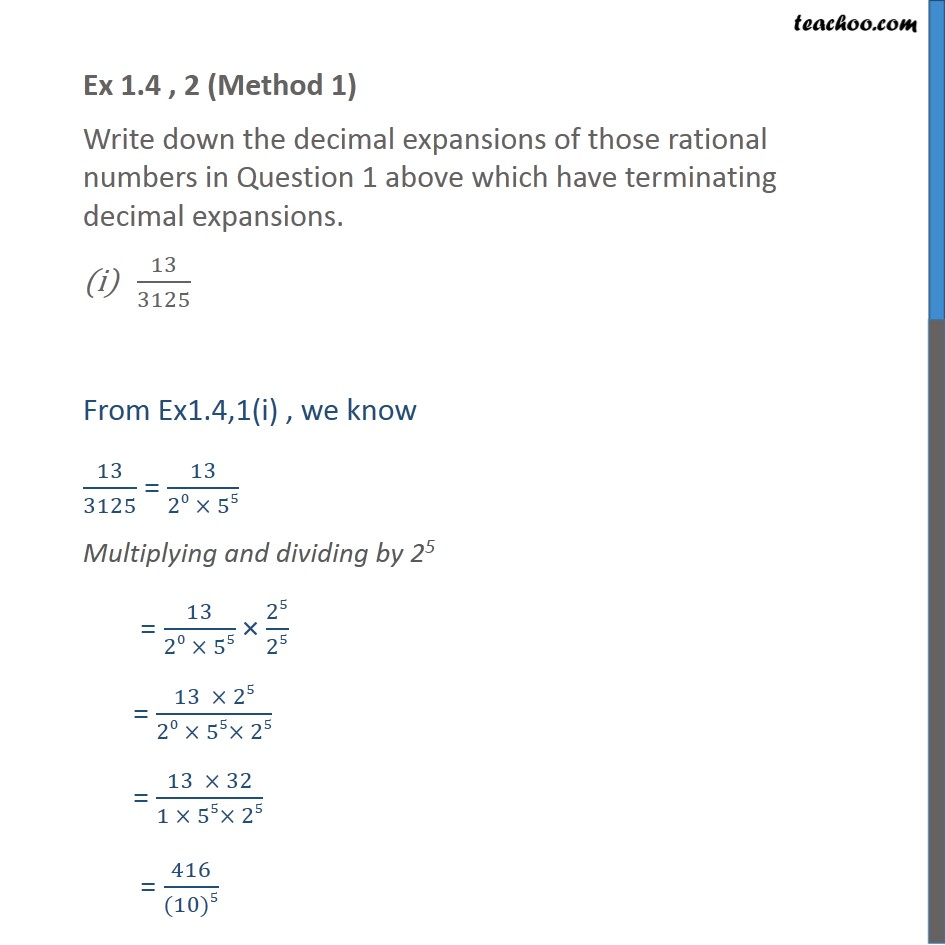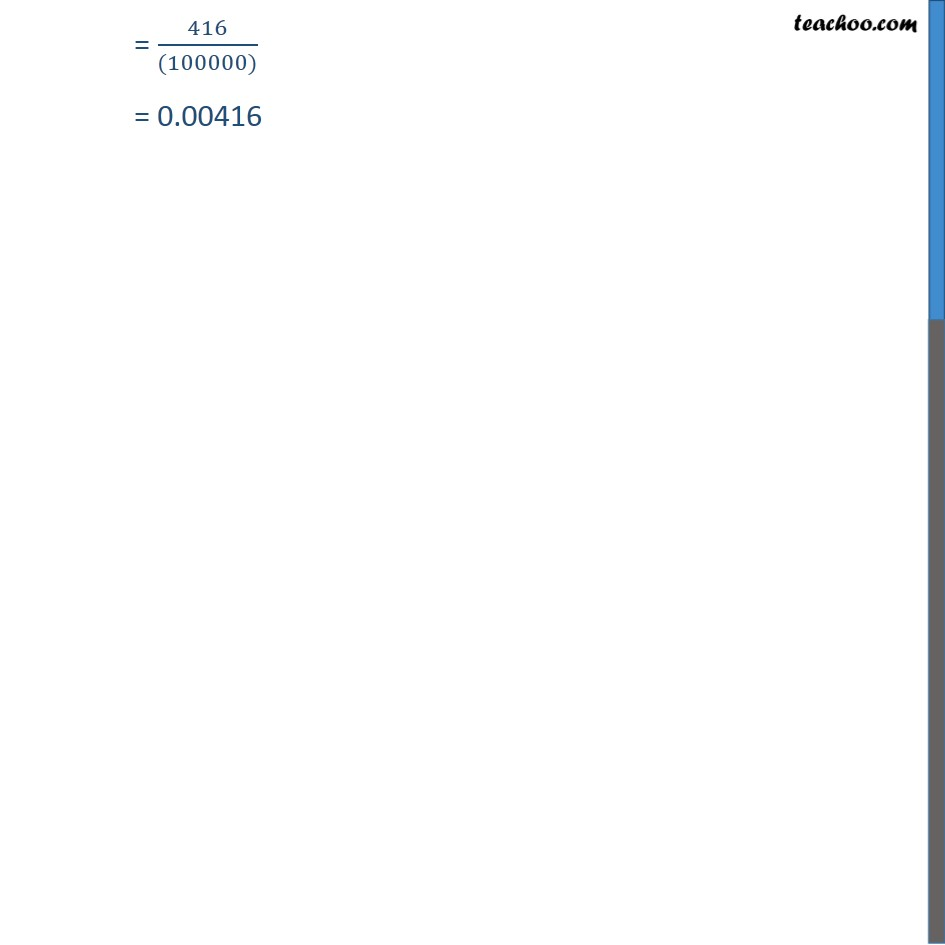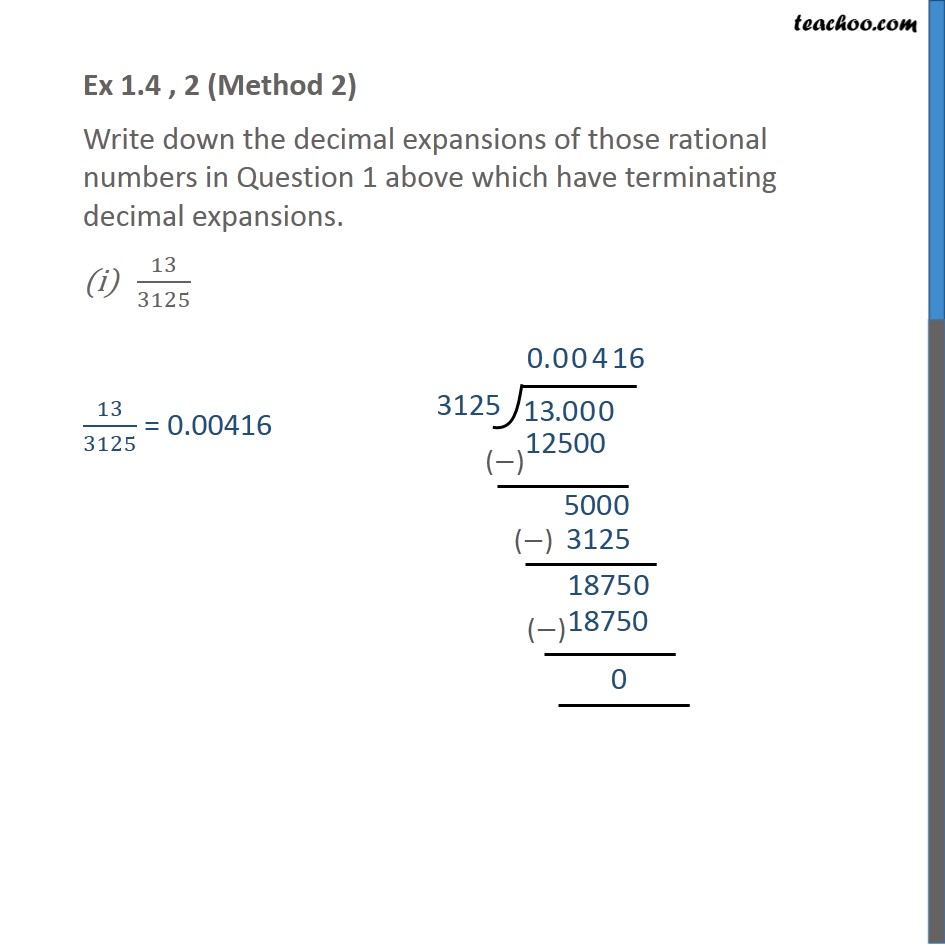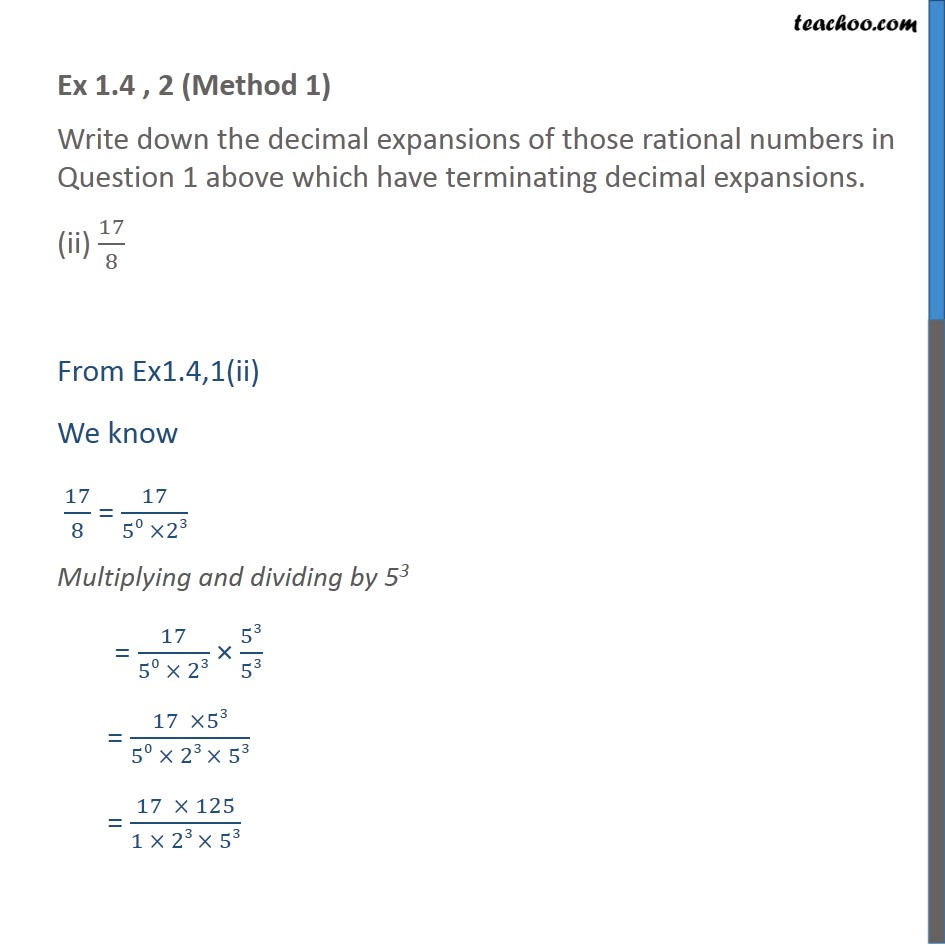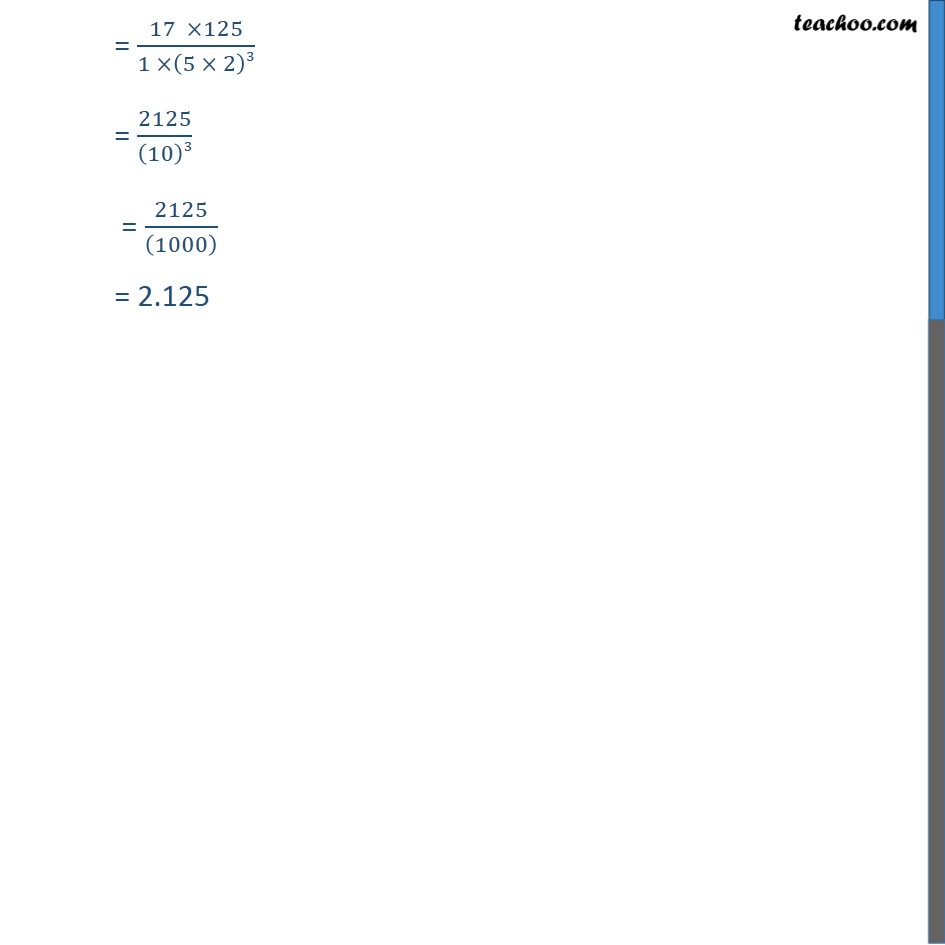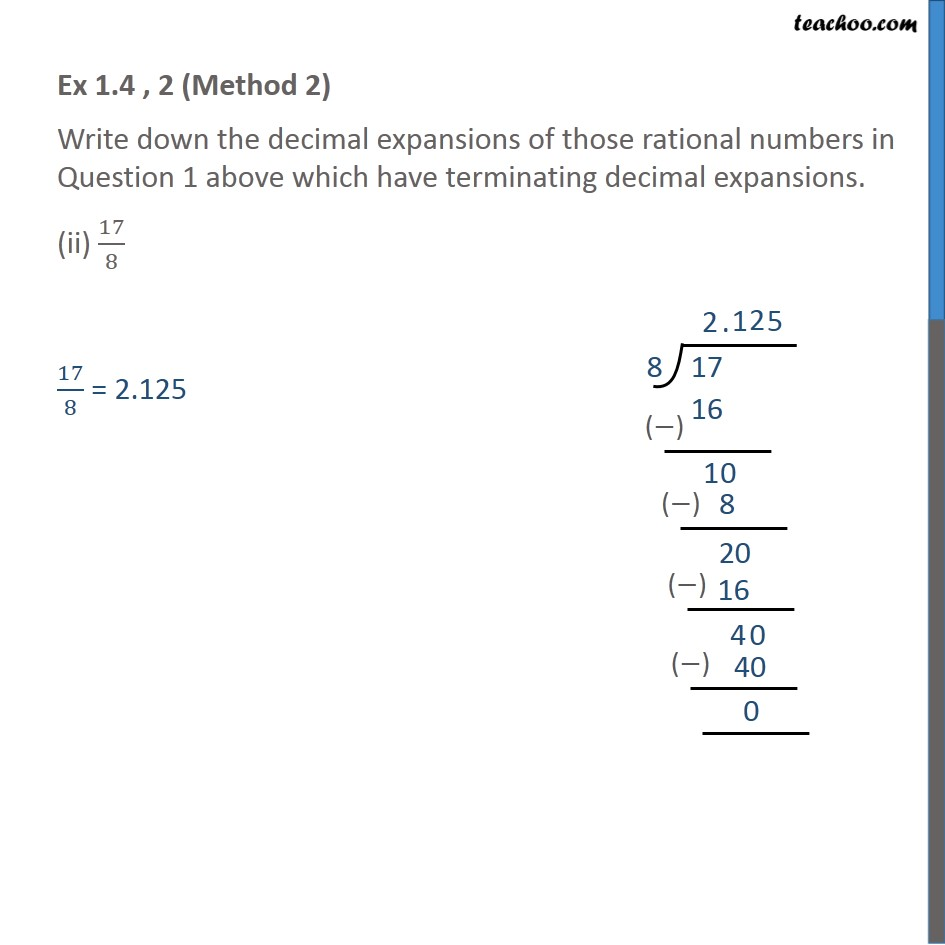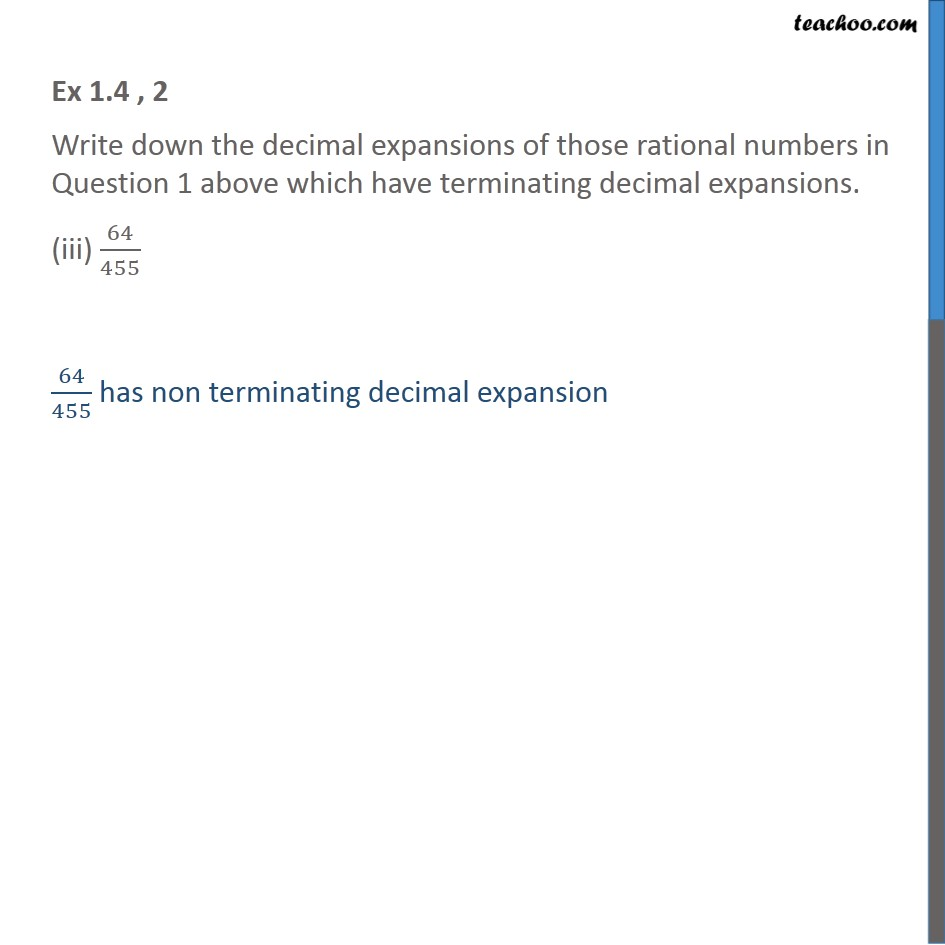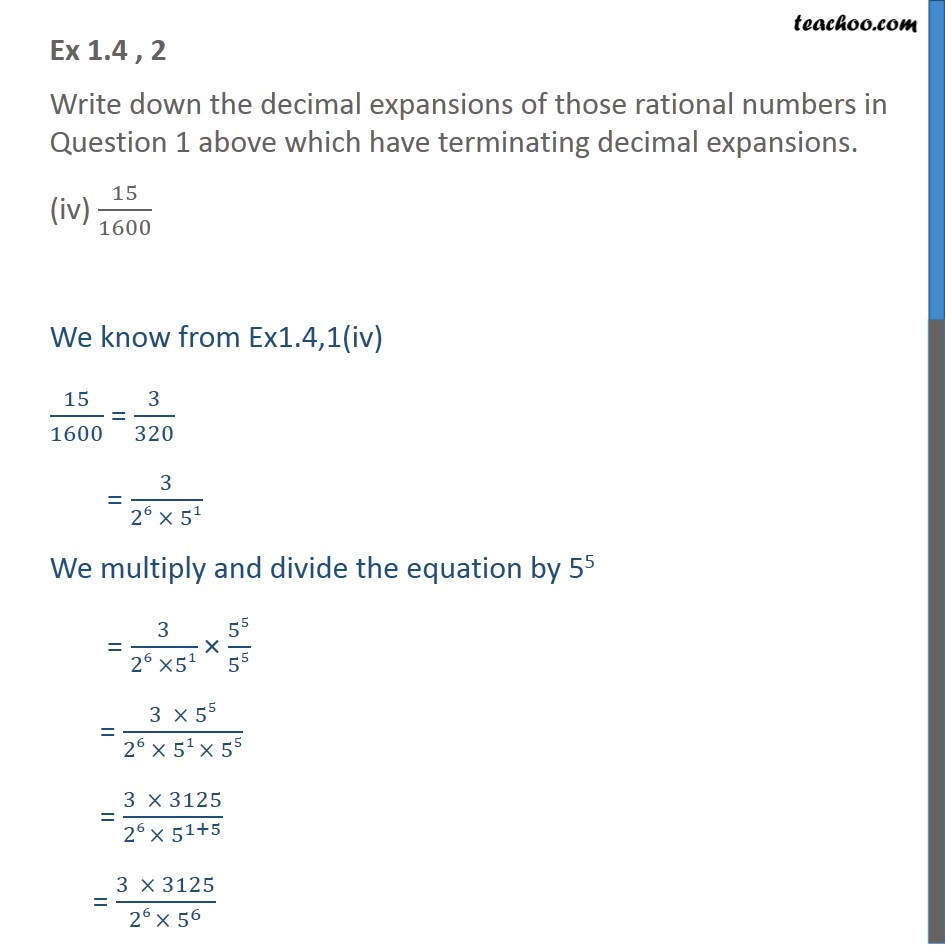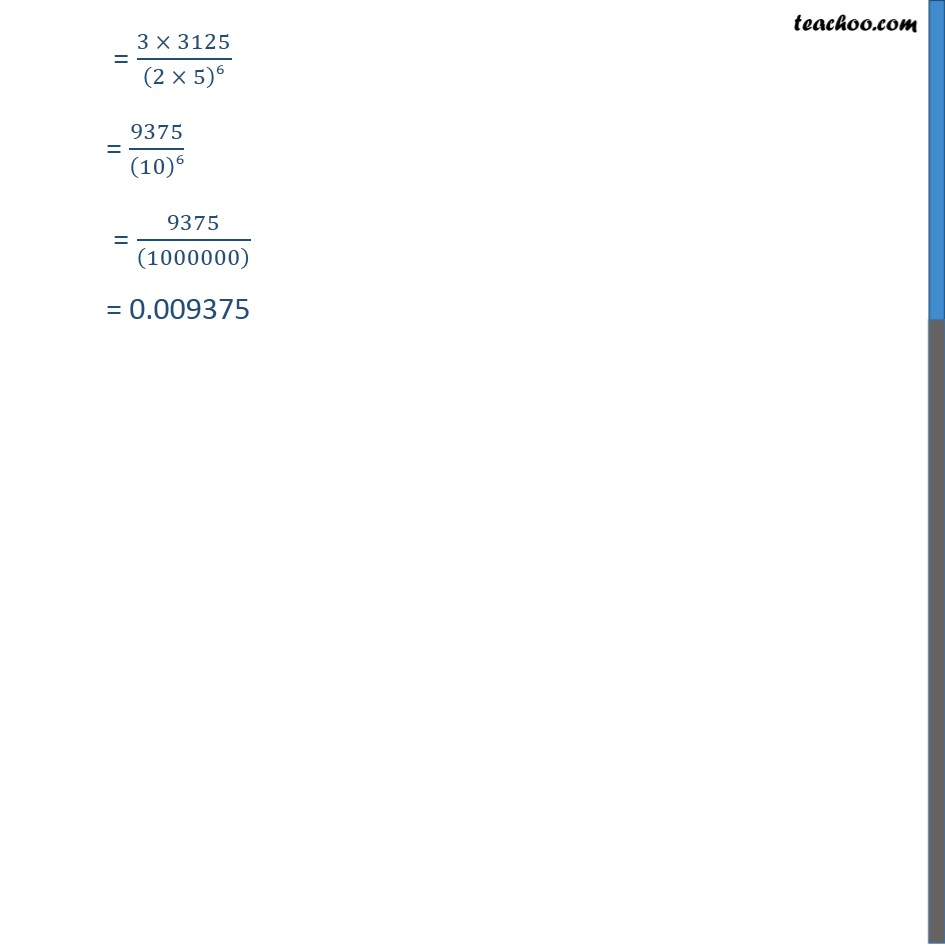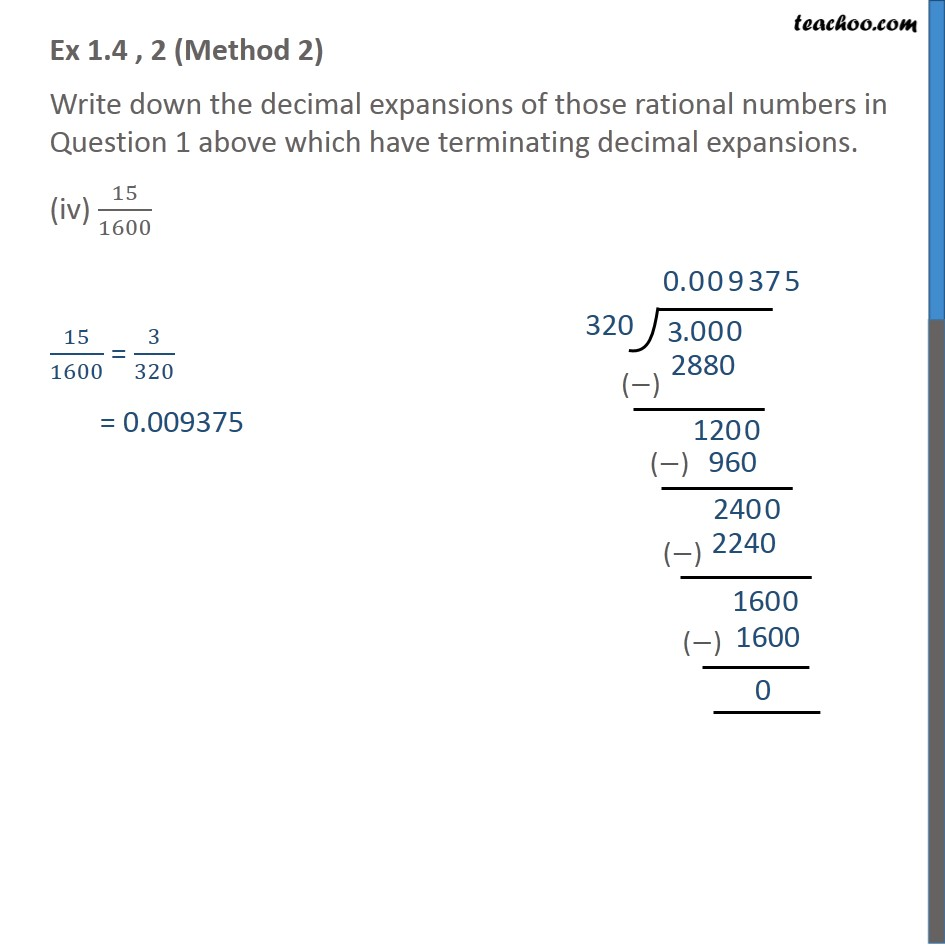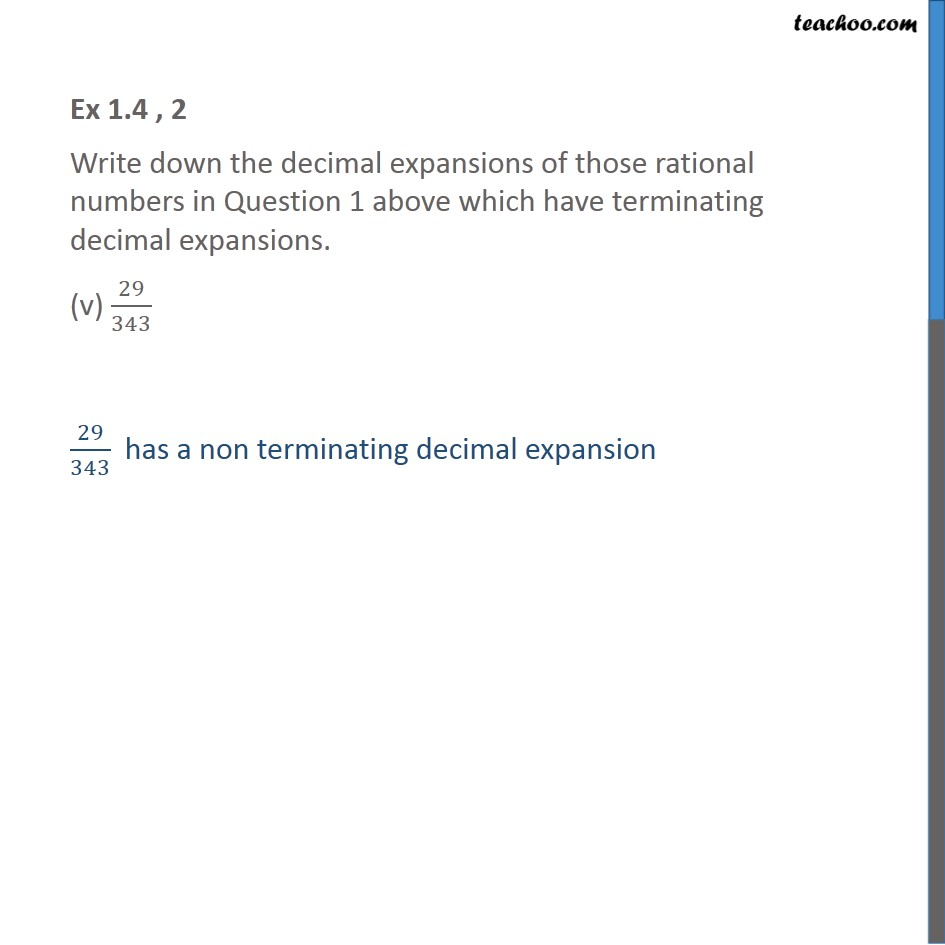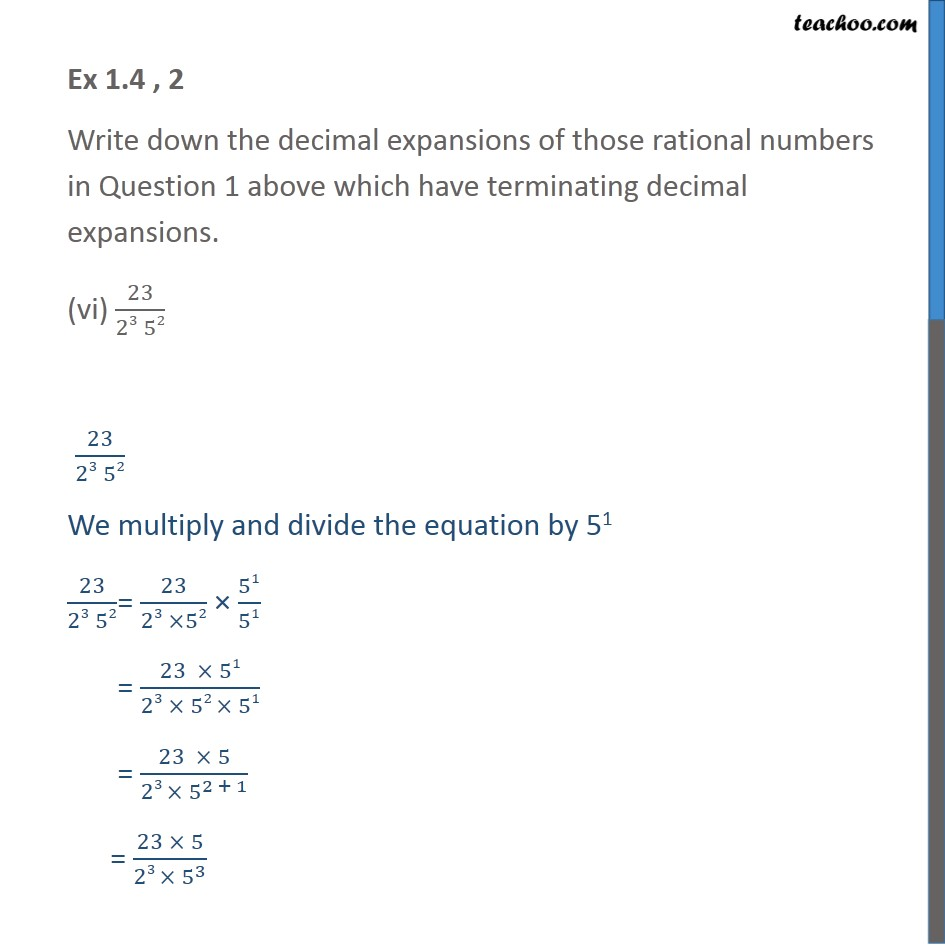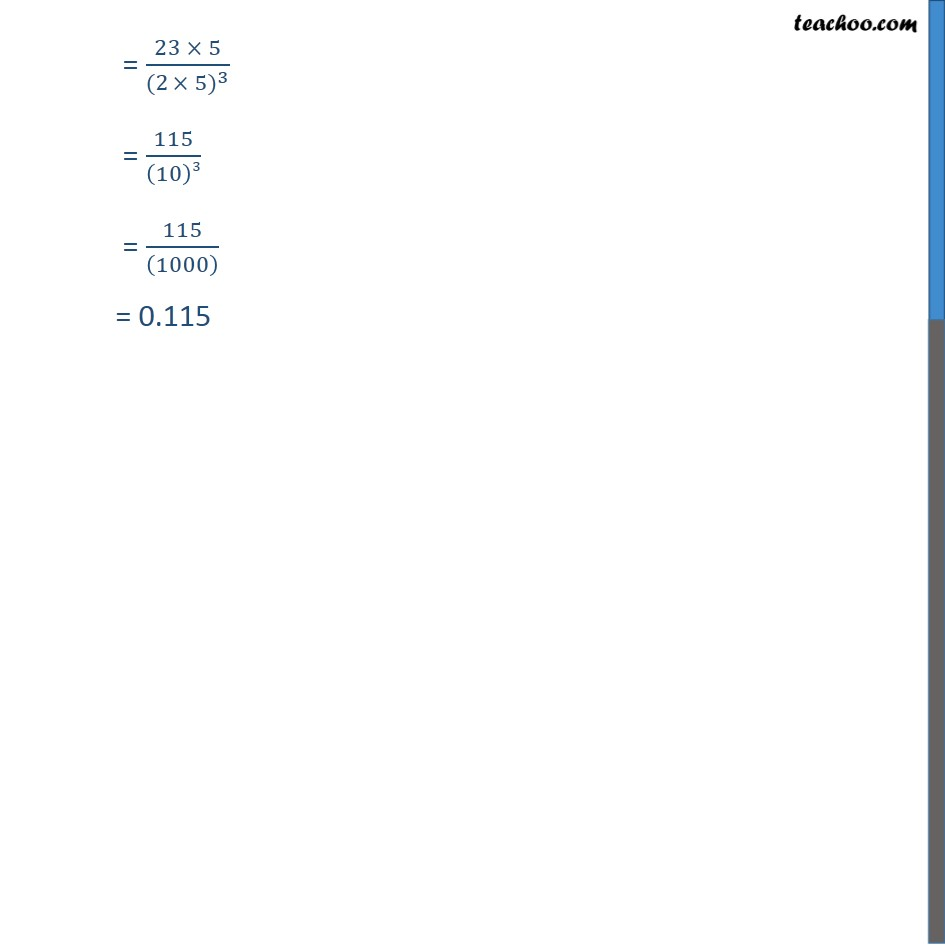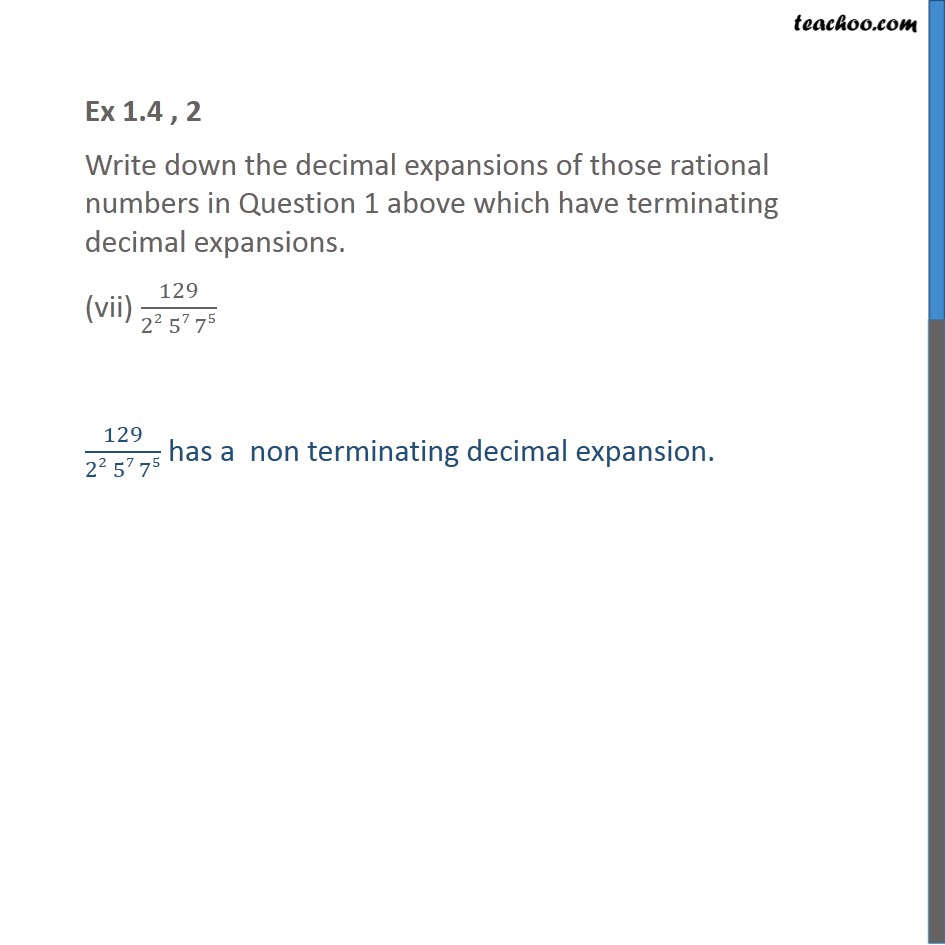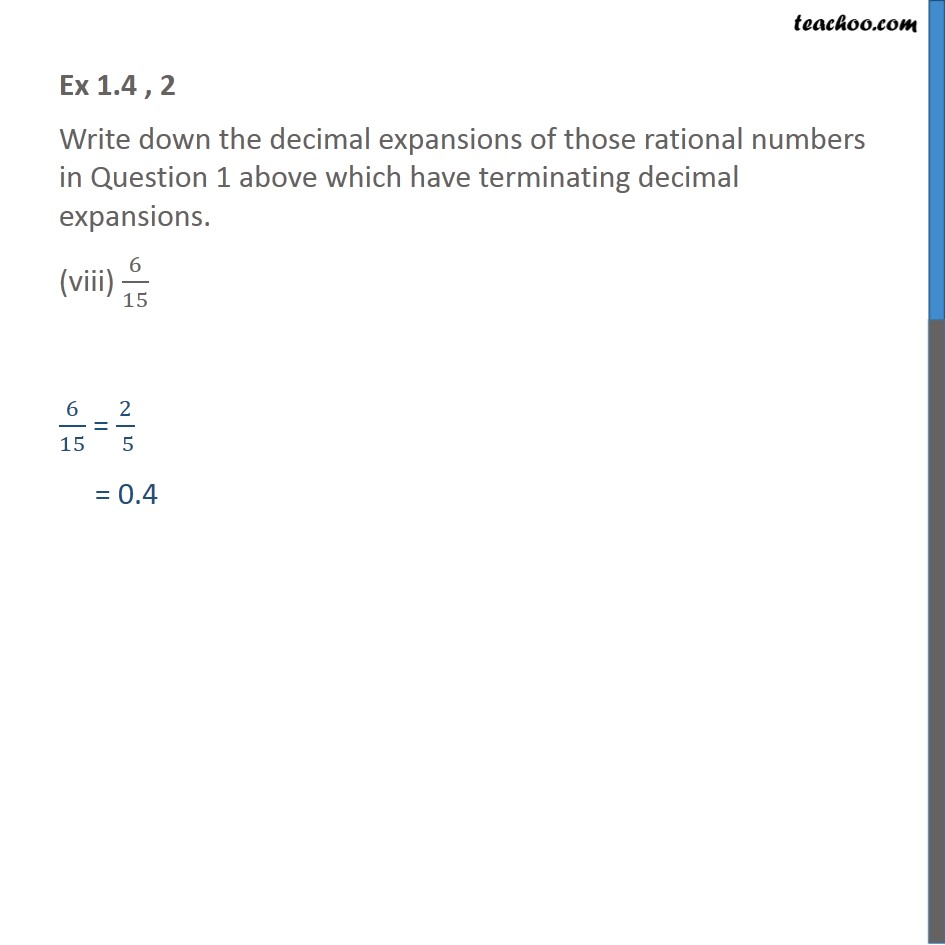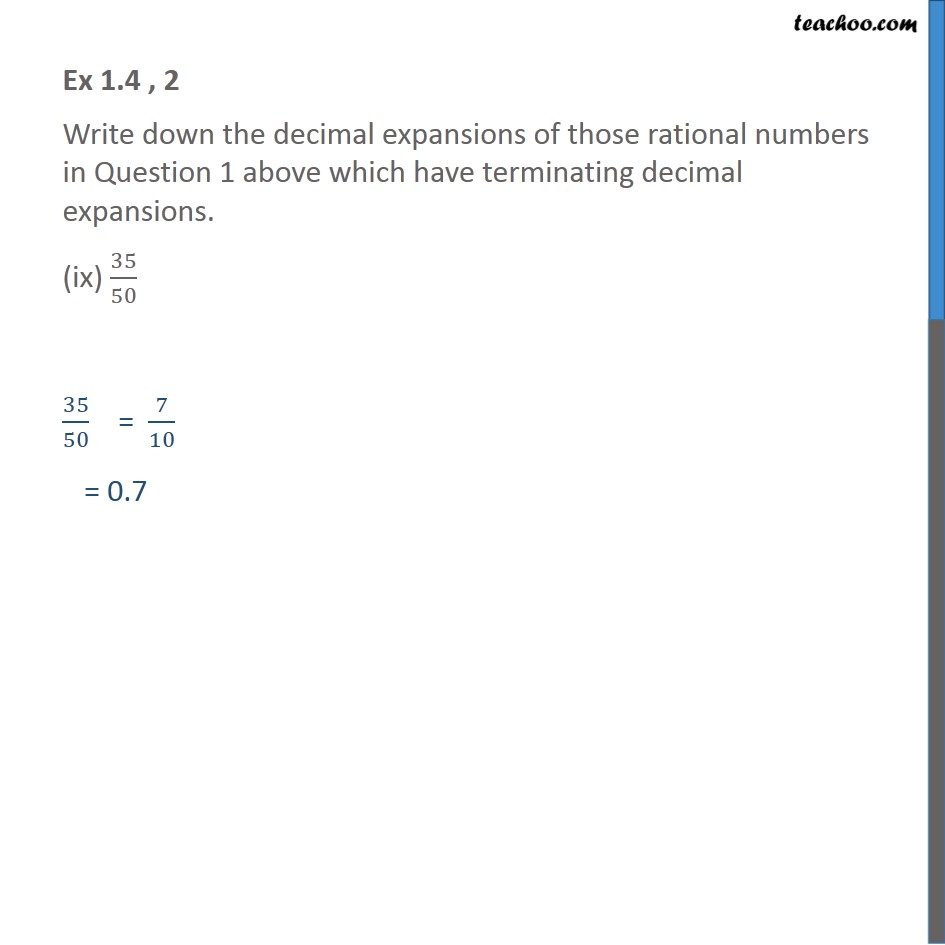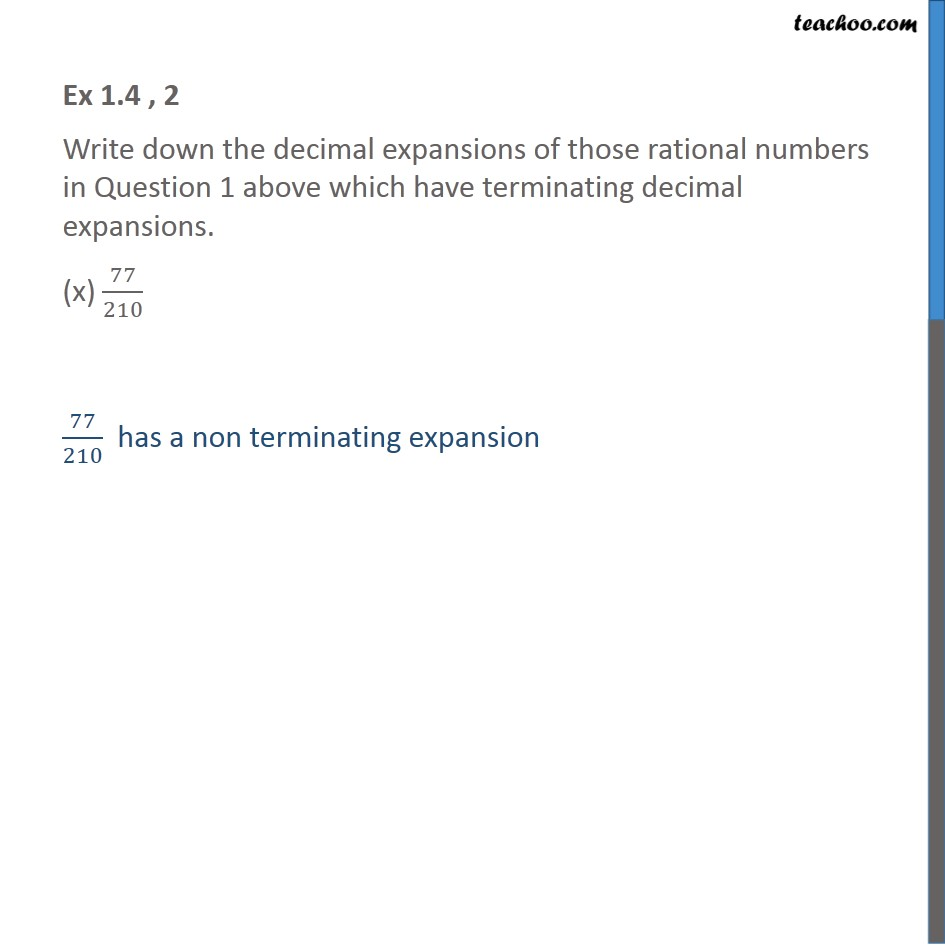1. Chapter 1 Class 10 Real Numbers
2. Concept wise
3. Decimal Expansion

Transcript

Ex 1.4 , 2 (Method 1) Write down the decimal expansions of those rational numbers in Question 1 above which have terminating decimal expansions. 13/3125 From Ex1.4,1(i) , we know 13/3125 = 13/(20 × 55) Multiplying and dividing by 25 = 13/(20 × 55) × 25/25 = (13 × 25)/(20 × 55× 25) = (13 × 32)/(1 × 55× 25) = 416/((10)5) = 416/((100000)) = 0.00416 Ex 1.4 , 2 (Method 2) Write down the decimal expansions of those rational numbers in Question 1 above which have terminating decimal expansions. 13/3125 13/3125 = 0.00416 Ex 1.4 , 2 (Method 1) Write down the decimal expansions of those rational numbers in Question 1 above which have terminating decimal expansions. (ii) 17/8 From Ex1.4,1(ii) We know 17/8 = 17/(50 ×23) Multiplying and dividing by 53 = 17/(50 × 23) × 53/53 = (17 ×53)/(50 × 23 × 53) = (17 × 125)/(1 × 23 × 53) = (17 ×125)/(1 ×(5 × 2)3) = 2125/(10)3 = 2125/((1000) ) = 2.125 Ex 1.4 , 2 (Method 2) Write down the decimal expansions of those rational numbers in Question 1 above which have terminating decimal expansions. (ii) 17/8 17/8 = 2.125 Ex 1.4 , 2 Write down the decimal expansions of those rational numbers in Question 1 above which have terminating decimal expansions. (iii) 64/455 64/455 has non terminating decimal expansion Ex 1.4 , 2 Write down the decimal expansions of those rational numbers in Question 1 above which have terminating decimal expansions. (iv) 15/1600 We know from Ex1.4,1(iv) 15/1600 = 3/320 = 3/(26 × 51) We multiply and divide the equation by 55 = 3/(26 ×51) × 55/55 = (3 × 55)/(26 × 51 × 55) = (3 × 3125)/(26 × 5^(1+5) ) = (3 × 3125)/(26 × 5^6 ) = (3 × 3125)/(2 × 5)6 = 9375/(10)6 = 9375/((1000000) ) = 0.009375 Ex 1.4 , 2 (Method 2) Write down the decimal expansions of those rational numbers in Question 1 above which have terminating decimal expansions. (iv) 15/1600 15/1600 = 3/320 = 0.009375 Ex 1.4 , 2 Write down the decimal expansions of those rational numbers in Question 1 above which have terminating decimal expansions. (v) 29/343 29/343 has a non terminating decimal expansion Ex 1.4 , 2 Write down the decimal expansions of those rational numbers in Question 1 above which have terminating decimal expansions. (vi) 23/(23 52) 23/(23 52) We multiply and divide the equation by 51 23/(23 52)= 23/(23 ×52) × 51/51 = (23 × 51)/(23 × 52 × 51) = (23 × 5)/(23 × 5^(2 + 1) ) = (23 × 5)/(23 × 5^3 ) = (23 × 5)/((2 × 〖5)〗^3 ) = 115/(10)3 = 115/((1000) ) = 0.115 Ex 1.4 , 2 Write down the decimal expansions of those rational numbers in Question 1 above which have terminating decimal expansions. (vii) 129/(22 57 75) 129/(22 57 75) has a non terminating decimal expansion. Ex 1.4 , 2 Write down the decimal expansions of those rational numbers in Question 1 above which have terminating decimal expansions. (viii) 6/15 6/15 = 2/( 5) = 0.4 Ex 1.4 , 2 Write down the decimal expansions of those rational numbers in Question 1 above which have terminating decimal expansions. (ix) 35/50 35/50 = 7/10 = 0.7 Ex 1.4 , 2 Write down the decimal expansions of those rational numbers in Question 1 above which have terminating decimal expansions. (x) 77/210 77/210 has a non terminating expansion

Decimal Expansion